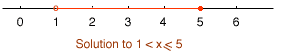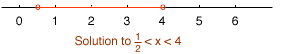Quandaries and Queries Name: cynthia Question: When graphing the solutions of an inequality, what is the difference between an open dot and a closed dot? Give an example. Hi Cynthia, Suppose that you want to plot the solution to the inequality 1< x ≤ 5 To do this you would draw a line segment from 1 to 5 but somehow, on the graph, you need to show that x = 5 satisfies the inequality but x = 1 does not. We use the open and closed dots to make this distinction. I put a closed dot at the "5" end of the interval to indicate that x = 5 satisfies the inequality, and I put an open dot at the "1" end of the interval to indicate that x = 1 does not satisfy the inequality.And another examplePenny